# Reliability problems¶

The structural reliability benchmarking framework is an on-line documentation platform that contains all kind of reliability problems with their descriptions, implementation files, and visualization of the performance functions. By making this information publicly available, it is intended to stimulate a systematic and reproducible assessment of the accuracy and efficiency of currently available reliability methods and those to come in the future. Furthermore, the platform is envisaged as a dynamic environment in which you are encouraged to contribute and add new, interesting problems.

Note

For most problems the failure probabilities are calculated with numerical methods that converge to the exact solution. Due to the applied stopping criteria of these numerical methods you may attain slightly different results. The difference should be a small difference in the second decimal of the normal form (scientific notation) representation of the failure probability. The reliability index ($$\beta$$) is calculated from the failure probability ($$P_\mathrm{f}$$) the following way: $$\beta=-\Phi^{-1}(P_\mathrm{f})$$.

## RP8¶

Six-dimensional hyperplane.

Table 9 – Tutorial set.
set_id problem_id
-1 1

### Overview¶

Category Value
Type symbolic
Number of random variables 6
Failure probability, $$P_\mathrm{f}$$ $$7.84\cdot10^{-4}$$
Reliability index, $$\beta=-\Phi^{-1}(P_\mathrm{f})$$ 3.16
Number of performance functions 1
Reference [Xu2018]

### Performance function¶

(1)\eqalign{ & {g_{\mathrm{comp}}}({\bf X}) = X_1 + 2X_2 + 2X_3 + X_4 - 5X_5 - 5X_6 \cr & {g_{\mathrm{sys}}}({\bf X}) = g_{\mathrm{comp}}({\bf X}) \cr}

### Random variables¶

The parametrization of distributions follows that of in Distributions.

Variable Description Distribution $$\theta_1$$ $$\theta_2$$ $$\theta_3$$ $$\theta_4$$ Mean Std
$$X_1$$ NA Lognormal 4.783 0.09975     120.0 12.0
$$X_2$$ NA Lognormal 4.783 0.09975     120.0 12.0
$$X_3$$ NA Lognormal 4.783 0.09975     120.0 12.0
$$X_4$$ NA Lognormal 4.783 0.09975     120.0 12.0
$$X_5$$ NA Lognormal 3.892 0.198     50.0 10.0
$$X_6$$ NA Lognormal 3.669 0.198     40.0 8.0

The random variables are mutually independent.

### Visualization¶### Implementation¶

#### Python¶

gfun_8(x)[source]

Performance function for reliability problem 8.

Parameters: x (numpy.array of float(s)) – Values of independent variables: columns are the different parameters/random variables (x1, x2,…xn) and rows are different parameter/random variables sets for different calls. g_val_sys (numpy.array of float(s)) – Performance function value for the system. g_val_comp (numpy.array of float(s)) – Performance function value for each component. msg (str) – Accompanying diagnostic message, e.g. warning.

#### Matlab¶

See gfun_8.m on GitLab.

## RP14¶

Table 10 – Challenge set 1.
set_id problem_id
1 1

### Overview¶

Category Value
Type Symbolic
Number of random variables 5
Failure probability, $$P_\mathrm{f}$$ $$7.52\cdot10^{-3}$$
Reliability index, $$\beta=-\Phi^{-1}(P_\mathrm{f})$$ 2.42
Number of performance functions 1
Reference [Schueller2004]

### Performance function¶

(2)\eqalign{ & {g_{\mathrm{comp}}}({\bf X}) = {X_1} - {{32} \over {\pi X_2^3}} \cdot \root 2 \of {{{X_3^2X_4^2} \over {16}} + X_5^2} \cr & {g_{\mathrm{sys}}}({\bf X}) = {g_{\mathrm{comp}}}({\bf X}) \cr}

### Random variables¶

The parametrization of distributions follows that of in Distributions.

Variable Description Distribution $$\theta_1$$ $$\theta_2$$ $$\theta_3$$ $$\theta_4$$ Mean Std
$$X_1$$ NA Uniform 70.0 80.0     75.0 2.887
$$X_2$$ NA Normal 39.0 0.1     39.0 0.1
$$X_3$$ NA Gumbel-max 1342.0 272.9     1500.0 350.0
$$X_4$$ NA Normal 400.0 0.1     400.0 0.1
$$X_5$$ NA Normal 250000.0 35000.0     250000.0 35000.0

The random variables are mutually independent.

### Visualization¶### Implementation¶

#### Python¶

gfun_14(x)[source]

Performance function for reliability problem 14.

Parameters: x (numpy.array of float(s)) – Values of independent variables: columns are the different parameters/random variables (x1, x2,…xn) and rows are different parameter/random variables sets for different calls. g_val_sys (numpy.array of float(s)) – Performance function value for the system. g_val_comp (numpy.array of float(s)) – Performance function value for each component. msg (str) – Accompanying diagnostic message, e.g. warning.

## RP22¶

Quadratic function with mixed term, convex.

Table 11 – Tutorial set.
set_id problem_id
-1 2

### Overview¶

Category Value
Type symbolic
Number of random variables 2
Failure probability, $$P_\mathrm{f}$$ $$4.16\cdot10^{-3}$$
Reliability index, $$\beta=-\Phi^{-1}(P_\mathrm{f})$$ 2.64
Number of performance functions 1
Reference [Grooteman2011]

### Performance function¶

(3)\eqalign{ & {g({\bf X})} = 2.5-\frac{(X_1 + X_2)}{\sqrt{2}} + 0.1\cdot (X_1 - X_2)^2 \cr & {g_{\mathrm{sys}}}({\bf X}) = g_{\mathrm{comp}}({\bf X}) \cr}

### Random variables¶

The parametrization of distributions follows that of in Distributions.

Variable Description Distribution $$\theta_1$$ $$\theta_2$$ $$\theta_3$$ $$\theta_4$$ Mean Std
$$X_1$$ NA Normal 0.0 1.0     0.0 1.0
$$X_2$$ NA Normal 0.0 1.0     0.0 1.0

The random variables are mutually independent.

### Visualization¶### Implementation¶

#### Python¶

gfun_22(x)[source]

Performance function for reliability problem 22.

Parameters: x (numpy.array of float(s)) – Values of independent variables: columns are the different parameters/random variables (x1, x2,…xn) and rows are different parameter/random variables sets for different calls. g_val_sys (numpy.array of float(s)) – Performance function value for the system. g_val_comp (numpy.array of float(s)) – Performance function value for each component. msg (str) – Accompanying diagnostic message, e.g. warning.

#### Matlab¶

See gfun_22.m on GitLab.

## RP24¶

Table 12 – Challenge set 1.
set_id problem_id
1 2

### Overview¶

Category Value
Type Symbolic
Number of random variables 2
Failure probability, $$P_\mathrm{f}$$ $$2.86\cdot10^{-3}$$
Reliability index, $$\beta=-\Phi^{-1}(P_\mathrm{f})$$ 2.76
Number of performance functions 1
Reference [Dai2016]

### Performance function¶

(4)\eqalign{ & {g_{\mathrm{comp}}}({\bf X}) = 2.5 - 0.2357 \cdot ({X_1} - {X_2}) + 0.00463 \cdot {({X_1} + {X_2} - 20)^4} \cr & {g_{\mathrm{sys}}}({\bf X}) = {g_{\mathrm{comp}}}({\bf X}) \cr}

### Random variables¶

The parametrization of distributions follows that of in Distributions.

Variable Description Distribution $$\theta_1$$ $$\theta_2$$ $$\theta_3$$ $$\theta_4$$ Mean Std
$$X_1$$ NA Normal 10.0 3.0     10.0 3.0
$$X_2$$ NA Normal 10.0 3.0     10.0 3.0

The random variables are mutually independent.

### Visualization¶### Implementation¶

#### Python¶

gfun_24(x)[source]

Performance function for reliability problem 22.

Parameters: x (numpy.array of float(s)) – Values of independent variables: columns are the different parameters/random variables (x1, x2,…xn) and rows are different parameter/random variables sets for different calls. g_val_sys (numpy.array of float(s)) – Performance function value for the system. g_val_comp (numpy.array of float(s)) – Performance function value for each component. msg (str) – Accompanying diagnostic message, e.g. warning.

## RP25¶

Table 13 – Challenge set 2.
set_id problem_id
2 1

### Overview¶

Category Value
Type Symbolic
Number of random variables 2
Failure probability, $$P_\mathrm{f}$$ $$6.14\cdot10^{-6}$$
Reliability index, $$\beta=-\Phi^{-1}(P_\mathrm{f})$$ 4.36
Number of performance functions 2
Reference [Dai2016]

### Performance function¶

(5)\eqalign{ & {g_{\mathrm{comp},1}}({\bf X}) = X_1^2 - 8 \cdot {X_2} + 16 \cr & {g_{\mathrm{comp},2}}({\bf X}) = - 16{X_1} + {X_2} + 32 \cr & {g_{\mathrm{sys}}}({\bf X}) = \max \left\{ \matrix{ {g_{\mathrm{comp},1}}{\bf X}) \hfill \cr {g_{\mathrm{comp},2}}({\bf X}) \hfill \cr} \right. \cr}

### Random variables¶

The parametrization of distributions follows that of in Distributions.

Variable Description Distribution $$\theta_1$$ $$\theta_2$$ $$\theta_3$$ $$\theta_4$$ Mean Std
$$X_{1}$$ NA Normal 0.0 1.0     0.0 1.0
$$X_{2}$$ NA Normal 0.0 1.0     0.0 1.0

The random variables are mutually independent.

### Visualization¶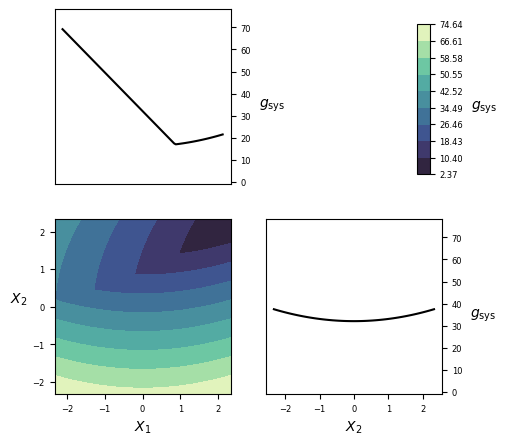### Implementation¶

#### Python¶

gfun_111(x)[source]

Performance function for reliability problem 111.

Parameters: x (numpy.array of float(s)) – Values of independent variables: columns are the different parameters/random variables (x1, x2,…xn) and rows are different parameter/random variables sets for different calls. g_val_sys (numpy.array of float(s)) – Performance function value for the system. g_val_comp (numpy.array of float(s)) – Performance function value for each component. msg (str) – Accompanying diagnostic message, e.g. warning.

## RP28¶

Table 14 – Challenge set 1.
set_id problem_id
1 3

### Overview¶

Category Value
Type Symbolic
Number of random variables 2
Failure probability, $$P_\mathrm{f}$$ $$1.46\cdot10^{-7}$$
Reliability index, $$\beta=-\Phi^{-1}(P_\mathrm{f})$$ 5.11
Number of performance functions 1
Reference [Dai2016]

### Performance function¶

(6)\eqalign{ & {g_{\mathrm{comp}}}({\bf X}) = {X_1} \cdot {X_2} - 146.14 \cr & {g_{\mathrm{sys}}}({\bf X}) = {g_{\mathrm{comp}}}({\bf X}) \cr}

### Random variables¶

The parametrization of distributions follows that of in Distributions.

Variable Description Distribution $$\theta_1$$ $$\theta_2$$ $$\theta_3$$ $$\theta_4$$ Mean Std
$$X_1$$ NA Normal 78064.0 11710.0     78064.0 11710.0
$$X_2$$ NA Normal 0.0104 0.00156     0.0104 0.00156

The random variables are mutually independent.

### Visualization¶### Implementation¶

#### Python¶

gfun_28(x)[source]

Performance function for reliability problem 28.

Parameters: x (numpy.array of float(s)) – Values of independent variables: columns are the different parameters/random variables (x1, x2,…xn) and rows are different parameter/random variables sets for different calls. g_val_sys (numpy.array of float(s)) – Performance function value for the system. g_val_comp (numpy.array of float(s)) – Performance function value for each component. msg (str) – Accompanying diagnostic message, e.g. warning.

## RP31¶

Table 15 – Challenge set 1.
set_id problem_id
1 4

### Overview¶

Category Value
Type Symbolic
Number of random variables 2
Failure probability, $$P_\mathrm{f}$$ $$1.80\cdot10^{-4}$$
Reliability index, $$\beta=-\Phi^{-1}(P_\mathrm{f})$$ 3.58
Number of performance functions 1
Reference [Schueller2004]

### Performance function¶

(7)\eqalign{ & {g_{\mathrm{comp}}}({\bf X}) = 2 - {X_2} + {(4 \cdot {X_1})^4} \cr & {g_{\mathrm{sys}}}({\bf X}) = {g_{\mathrm{comp}}}({\bf X}) \cr}

### Random variables¶

The parametrization of distributions follows that of in Distributions.

Variable Description Distribution $$\theta_1$$ $$\theta_2$$ $$\theta_3$$ $$\theta_4$$ Mean Std
$$X_1$$ NA Normal 0.0 1.0     0.0 1.0
$$X_2$$ NA Normal 0.0 1.0     0.0 1.0

The random variables are mutually independent.

### Visualization¶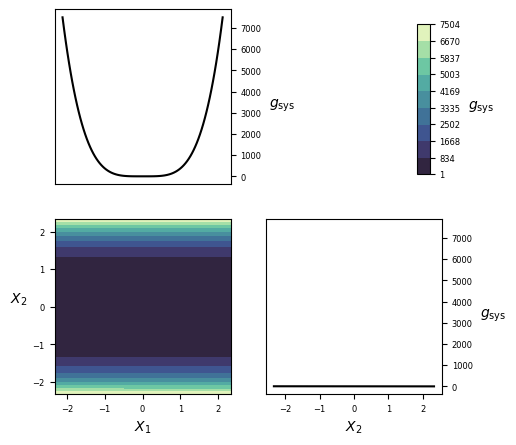### Implementation¶

#### Python¶

gfun_31(x)[source]

Performance function for reliability problem 31.

Parameters: x (numpy.array of float(s)) – Values of independent variables: columns are the different parameters/random variables (x1, x2,…xn) and rows are different parameter/random variables sets for different calls. g_val_sys (numpy.array of float(s)) – Performance function value for the system. g_val_comp (numpy.array of float(s)) – Performance function value for each component. msg (str) – Accompanying diagnostic message, e.g. warning.

## RP33¶

Table 16
set_id problem_id
-1 3
2 2

### Overview¶

Category Value
Type Symbolic
Number of random variables 3
Failure probability, $$P_\mathrm{f}$$ $$2.57\cdot10^{-3}$$
Reliability index, $$\beta=-\Phi^{-1}(P_\mathrm{f})$$ 2.80
Number of performance functions 2
Reference [Schueller2004]

### Performance function¶

(8)\eqalign{ & {g_{\mathrm{comp},1}}({\bf X}) = - {X_1} - {X_2} - {X_3} + 3 \cdot \root 2 \of 3 \cr & {g_{\mathrm{comp},2}}\left( {\bf X} \right) = - {X_3} + 3 \cr & {g_{\mathrm{sys}}}({\bf X}) = \min \left\{ \matrix{ {g_{\mathrm{comp},1}}({\bf X}) \hfill \cr {g_{\mathrm{comp},2}}\left( {\bf X} \right) \hfill \cr} \right. \cr}

### Random variables¶

The parametrization of distributions follows that of in Distributions.

Variable Description Distribution $$\theta_1$$ $$\theta_2$$ $$\theta_3$$ $$\theta_4$$ Mean Std
$$X_{1}$$ NA Normal 0.0 1.0     0.0 1.0
$$X_{2}$$ NA Normal 0.0 1.0     0.0 1.0
$$X_{3}$$ NA Normal 0.0 1.0     0.0 1.0

The random variables are mutually independent.

### Visualization¶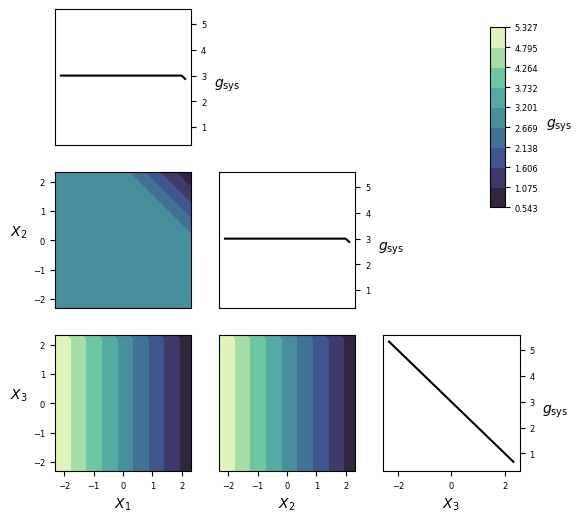### Implementation¶

gfun_33(x)[source]

Performance function for reliability problem 33.

Parameters: x (numpy.array of float(s)) – Values of independent variables: columns are the different parameters/random variables (x1, x2,…xn) and rows are different parameter/random variables sets for different calls. g_val_sys (numpy.array of float(s)) – Performance function value for the system. g_val_comp (numpy.array of float(s)) – Performance function value for each component. msg (str) – Accompanying diagnostic message, e.g. warning.

## RP35¶

Table 17 – Challenge set 2.
set_id problem_id
2 3

### Overview¶

Category Value
Type Symbolic
Number of random variables 2
Failure probability, $$P_\mathrm{f}$$ $$3.54\cdot10^{-3}$$
Reliability index, $$\beta=-\Phi^{-1}(P_\mathrm{f})$$ 2.70
Number of performance functions 2
Reference [Dai2016]

### Performance function¶

(9)\eqalign{ & {g_{\mathrm{comp},1}}({\bf X}) = 2 - {X_2} + \exp ( - 0.1 \cdot X_1^2) + {(0.2 \cdot {X_1})^4} \cr & {g_{\mathrm{comp},2}}({\bf X}) = 4.5 - {X_1} \cdot {X_2} \cr & {g_{\mathrm{sys}}}({\bf X}) = \min \left\{ \matrix{ {g_{\mathrm{comp},1}}({\bf X}) \hfill \cr {g_{\mathrm{comp},2}}({\bf X}) \hfill \cr} \right. \cr}

### Random variables¶

The parametrization of distributions follows that of in Distributions.

Variable Description Distribution $$\theta_1$$ $$\theta_2$$ $$\theta_3$$ $$\theta_4$$ Mean Std
$$X_{1}$$ NA Normal 0.0 1.0     0.0 1.0
$$X_{2}$$ NA Normal 0.0 1.0     0.0 1.0

The random variables are mutually independent.

### Visualization¶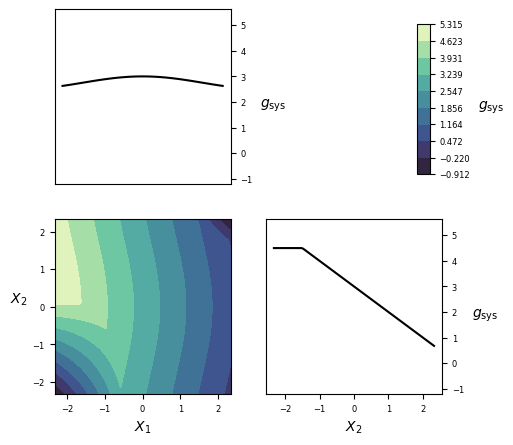### Implementation¶

gfun_35(x)[source]

Performance function for reliability problem 35.

Parameters: x (numpy.array of float(s)) – Values of independent variables: columns are the different parameters/random variables (x1, x2,…xn) and rows are different parameter/random variables sets for different calls. g_val_sys (numpy.array of float(s)) – Performance function value for the system. g_val_comp (numpy.array of float(s)) – Performance function value for each component. msg (str) – Accompanying diagnostic message, e.g. warning.

## RP38¶

Table 18 – Challenge set 1.
set_id problem_id
1 5

### Overview¶

Category Value
Type Symbolic
Number of random variables 7
Failure probability, $$P_\mathrm{f}$$ $$8.10\cdot10^{-3}$$
Reliability index, $$\beta=-\Phi^{-1}(P_\mathrm{f})$$ 2.48
Number of performance functions 1
Reference [Schueller2004]

### Performance function¶

(10)\eqalign{ & {g_{\mathrm{comp}}}({\bf X}) = 15.59 \cdot {10^4} - {{{X_1} \cdot X_2^3} \over {2 \cdot X_2^3}} \cdot {{X_4^2 - 4 \cdot {X_5} \cdot {X_6} \cdot X_7^2 + {X_4} \cdot ({X_6} + 4 \cdot {X_5} + 2 \cdot {X_6} \cdot {X_7})} \over {{X_4} \cdot {X_5} \cdot ({X_4} + {X_6} + 2 \cdot {X_6} \cdot {X_7})}} \cr & {g_{\mathrm{sys}}}({\bf X}) = {g_{\mathrm{comp}}}({\bf X}) \cr}

### Random variables¶

The parametrization of distributions follows that of in Distributions.

Variable Description Distribution $$\theta_1$$ $$\theta_2$$ $$\theta_3$$ $$\theta_4$$ Mean Std
$$X_1$$ NA Normal 350.0 35.0     350.0 35.0
$$X_2$$ NA Normal 50.8 5.08     50.8 5.08
$$X_3$$ NA Normal 3.81 0.381     3.81 0.381
$$X_4$$ NA Normal 173.0 17.3     173.0 17.3
$$X_5$$ NA Normal 9.38 0.938     9.38 0.938
$$X_6$$ NA Normal 33.1 3.31     33.1 3.31
$$X_7$$ NA Normal 0.036 0.0036     0.036 0.0036

The random variables are mutually independent.

### Visualization¶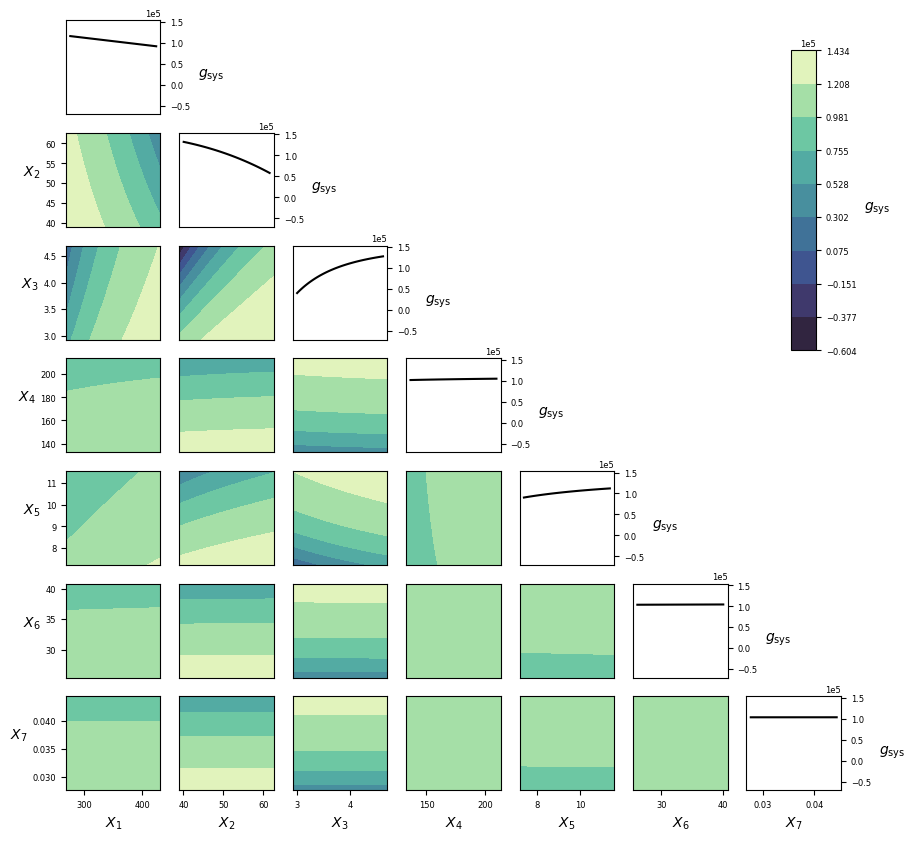### Implementation¶

gfun_38(x)[source]

Performance function for reliability problem 38.

Parameters: x (numpy.array of float(s)) – Values of independent variables: columns are the different parameters/random variables (x1, x2,…xn) and rows are different parameter/random variables sets for different calls. g_val_sys (numpy.array of float(s)) – Performance function value for the system. g_val_comp (numpy.array of float(s)) – Performance function value for each component. msg (str) – Accompanying diagnostic message, e.g. warning.

## RP53¶

Table 19 – Challenge set 1.
set_id problem_id
1 6

### Overview¶

Category Value
Type Symbolic
Number of random variables 2
Failure probability, $$P_\mathrm{f}$$ $$3.13\cdot10^{-2}$$
Reliability index, $$\beta=-\Phi^{-1}(P_\mathrm{f})$$ 1.86
Number of performance functions 1
Reference [Schueller2004]

### Performance function¶

(11)\eqalign{ & {g_{\mathrm{comp}}}({\bf X}) = \sin \left( {{{5 \cdot {X_1}} \over 2}} \right) + 2 - {{(X_1^2 + 4) \cdot ({X_2} - 1)} \over {20}} \cr & {g_{\mathrm{sys}}}({\bf X}) = {g_{\mathrm{comp}}}({\bf X}) \cr}

### Random variables¶

The parametrization of distributions follows that of in Distributions.

Variable Description Distribution $$\theta_1$$ $$\theta_2$$ $$\theta_3$$ $$\theta_4$$ Mean Std
$$X_1$$ NA Normal 1.5 1.0     1.5 1.0
$$X_2$$ NA Normal 2.5 1.0     2.5 1.0

The random variables are mutually independent.

### Visualization¶### Implementation¶

#### Python¶

gfun_53(x)[source]

Performance function for reliability problem 53.

Parameters: x (numpy.array of float(s)) – Values of independent variables: columns are the different parameters/random variables (x1, x2,…xn) and rows are different parameter/random variables sets for different calls. g_val_sys (numpy.array of float(s)) – Performance function value for the system. g_val_comp (numpy.array of float(s)) – Performance function value for each component. msg (str) – Accompanying diagnostic message, e.g. warning.

## RP54¶

Table 20 – Challenge set 1.
set_id problem_id
1 7

### Overview¶

Category Value
Type Symbolic
Number of random variables 20
Failure probability, $$P_\mathrm{f}$$ $$9.98\cdot10^{-4}$$
Reliability index, $$\beta=-\Phi^{-1}(P_\mathrm{f})$$ 3.09
Number of performance functions 1
Reference [Dai2016]

### Performance function¶

(12)\eqalign{ & {g_{\mathrm{comp}}}({\bf X}) = \sum\limits_{i = 1}^{20} {{X_i} - 8.951} \cr & {g_{\mathrm{sys}}}({\bf X}) = {g_{\mathrm{comp}}}({\bf X}) \cr}

### Random variables¶

The parametrization of distributions follows that of in Distributions.

Variable Description Distribution $$\theta_1$$ $$\theta_2$$ $$\theta_3$$ $$\theta_4$$ Mean Std
$$X_1$$ NA Exponential 1.0       1.0 1.0
$$\vdots$$ $$\vdots$$ $$\vdots$$ $$\vdots$$ $$\vdots$$ $$\vdots$$ $$\vdots$$ $$\vdots$$ $$\vdots$$
$$X_{20}$$ NA Exponential 1.0       1.0 1.0

The random variables are identically distributed and mutually independent.

### Visualization¶### Implementation¶

gfun_54(x)[source]

Performance function for reliability problem 54.

Parameters: x (numpy.array of float(s)) – Values of independent variables: columns are the different parameters/random variables (x1, x2,…xn) and rows are different parameter/random variables sets for different calls. g_val_sys (numpy.array of float(s)) – Performance function value for the system. g_val_comp (numpy.array of float(s)) – Performance function value for each component. msg (str) – Accompanying diagnostic message, e.g. warning.

## RP55¶

Table 21 – Challenge set 2.
set_id problem_id
2 4

### Overview¶

Category Value
Type Symbolic
Number of random variables 2
Failure probability, $$P_\mathrm{f}$$ $$3.60\cdot10^{-1}$$
Reliability index, $$\beta=-\Phi^{-1}(P_\mathrm{f})$$ -0.15
Number of performance functions 4
Reference [Schueller2004]

### Performance function¶

(13)\eqalign{ & {g_{\mathrm{comp},1}}({\bf X}) = 0.2 + 0.6 \cdot {({X_1} - {X_2})^4} - {{{X_1} - {X_2}} \over {\root 2 \of 2 }} \cr & {g_{\mathrm{comp},2}}({\bf X}) = 0.2 + 0.6 \cdot {({X_1} - {X_2})^4} + {{{X_1} - {X_2}} \over {\root 2 \of 2 }} \cr & {g_{\mathrm{comp},3}}({\bf X}) = {X_1} - {X_2} + {5 \over {\root 2 \of 2 }} - 2.2 \cr & {g_{\mathrm{comp},4}}({\bf X}) = {X_2} - {X_1} + {5 \over {\root 2 \of 2 }} - 2.2 \cr & {g_{\mathrm{sys}}}({\bf X}) = \min \left\{ \matrix{ {g_{\mathrm{comp},1}}({\bf X}) \hfill \cr {g_{\mathrm{comp},2}}({\bf X}) \hfill \cr {g_{\mathrm{comp},3}}({\bf X}) \hfill \cr {g_{\mathrm{comp},4}}({\bf X}) \hfill \cr} \right. \cr}

### Random variables¶

The parametrization of distributions follows that of in Distributions.

Variable Description Distribution $$\theta_1$$ $$\theta_2$$ $$\theta_3$$ $$\theta_4$$ Mean Std
$$X_{1}$$ NA Uniform -1.0 1.0     0.0 0.5774
$$X_{2}$$ NA Uniform -1.0 1.0     0.0 0.5774

The random variables are mutually independent.

### Visualization¶### Implementation¶

#### Python¶

gfun_55(x)[source]

Performance function for reliability problem 55.

Parameters: x (numpy.array of float(s)) – Values of independent variables: columns are the different parameters/random variables (x1, x2,…xn) and rows are different parameter/random variables sets for different calls. g_val_sys (numpy.array of float(s)) – Performance function value for the system. g_val_comp (numpy.array of float(s)) – Performance function value for each component. msg (str) – Accompanying diagnostic message, e.g. warning.

## RP57¶

Table 22 – Challenge set 2.
set_id problem_id
2 5

### Overview¶

Category Value
Type Symbolic
Number of random variables 2
Failure probability, $$P_\mathrm{f}$$ $$2.84\cdot10^{-2}$$
Reliability index, $$\beta=-\Phi^{-1}(P_\mathrm{f})$$ 1.91
Number of performance functions 3
Reference [Schueller2004]

### Performance function¶

(14)\eqalign{ & {g_{\mathrm{comp},1}}({\bf X}) = - X_1^2 + X_2^3 + 3 \cr & {g_{\mathrm{comp},2}}({\bf X}) = 2 - {X_1} - 8 \cdot {X_2} \cr & {g_{\mathrm{comp},3}}({\bf X}) = {({X_1} + 3)^2} + {({X_2} + 3)^2} - 4 \cr & {g_{\mathrm{sys}}}({\bf X}) = \min \left\{ \matrix{ \max \left\{ \matrix{ {g_{\mathrm{comp},1}}({\bf X}) \hfill \cr {g_{\mathrm{comp},2}}({\bf X}) \hfill \cr} \right. \hfill \cr {g_{\mathrm{comp},3}}({\bf X}) \hfill \cr} \right. \cr}

### Random variables¶

The parametrization of distributions follows that of in Distributions.

Variable Description Distribution $$\theta_1$$ $$\theta_2$$ $$\theta_3$$ $$\theta_4$$ Mean Std
$$X_{1}$$ NA Normal 0.0 1.0     0.0 1.0
$$X_{2}$$ NA Normal 0.0 1.0     0.0 1.0

The random variables are mutually independent.

### Visualization¶### Implementation¶

#### Python¶

gfun_57(x)[source]

Performance function for reliability problem 57.

Parameters: x (numpy.array of float(s)) – Values of independent variables: columns are the different parameters/random variables (x1, x2,…xn) and rows are different parameter/random variables sets for different calls. g_val_sys (numpy.array of float(s)) – Performance function value for the system. g_val_comp (numpy.array of float(s)) – Performance function value for each component. msg (str) – Accompanying diagnostic message, e.g. warning.

## RP60¶

Table 23 – Challenge set 2.
set_id problem_id
2 6

### Overview¶

Category Value
Type Symbolic
Number of random variables 5
Failure probability, $$P_\mathrm{f}$$ $$4.56\cdot10^{-2}$$
Reliability index, $$\beta=-\Phi^{-1}(P_\mathrm{f})$$ 1.70
Number of performance functions 7
Reference [Schueller2004]

### Performance function¶

(15)\eqalign{ & {g_{\mathrm{comp},1}}({\bf X}) = {X_1} - {X_5} \cr & {g_{\mathrm{comp},2}}({\bf X}) = {X_2} - {{{X_5}} \over 2} \cr & {g_{\mathrm{comp},3}}({\bf X}) = {X_3} - {{{X_5}} \over 2} \cr & {g_{\mathrm{comp},4}}({\bf X}) = {X_4} - {{{X_5}} \over 2} \cr & {g_{\mathrm{comp},5}}({\bf X}) = {X_2} - {X_5} \cr & {g_{\mathrm{comp},6}}({\bf X}) = {X_3} - {X_5} \cr & {g_{\mathrm{comp},7}}({\bf X}) = {X_4} - {X_5} \cr & {g_{\mathrm{sys}}}({\bf X}) = \min \left\{ \matrix{ {g_{\mathrm{comp},1}}({\bf X}) \hfill \cr \max \left\{ \matrix{ \min \left\{ \matrix{ {g_{\mathrm{comp},2}}({\bf X}) \hfill \cr {g_{\mathrm{comp},3}}({\bf X}) \hfill \cr {g_{\mathrm{comp},4}}({\bf X}) \hfill \cr} \right. \hfill \cr \max \left\{ \matrix{ \min \left\{ \matrix{ {g_{\mathrm{comp},5}}({\bf X}) \hfill \cr {g_{\mathrm{comp},6}}({\bf X}) \hfill \cr} \right. \hfill \cr {g_{\mathrm{comp},7}}({\bf X}) \hfill \cr} \right. \hfill \cr} \right. \hfill \cr} \right. \cr}

### Random variables¶

The parametrization of distributions follows that of in Distributions.

Variable Description Distribution $$\theta_1$$ $$\theta_2$$ $$\theta_3$$ $$\theta_4$$ Mean Std
$$X_{1}$$ NA Lognormal 7.691 0.09975     2200.0 220.0
$$X_{2}$$ NA Lognormal 7.645 0.09975     2100.0 210.0
$$X_{3}$$ NA Lognormal 7.736 0.09975     2300.0 230.0
$$X_{4}$$ NA Lognormal 7.596 0.09975     2000.0 200.0
$$X_{5}$$ NA Lognormal 7.016 0.3853     1200.0 480.0

The random variables are mutually independent.

### Visualization¶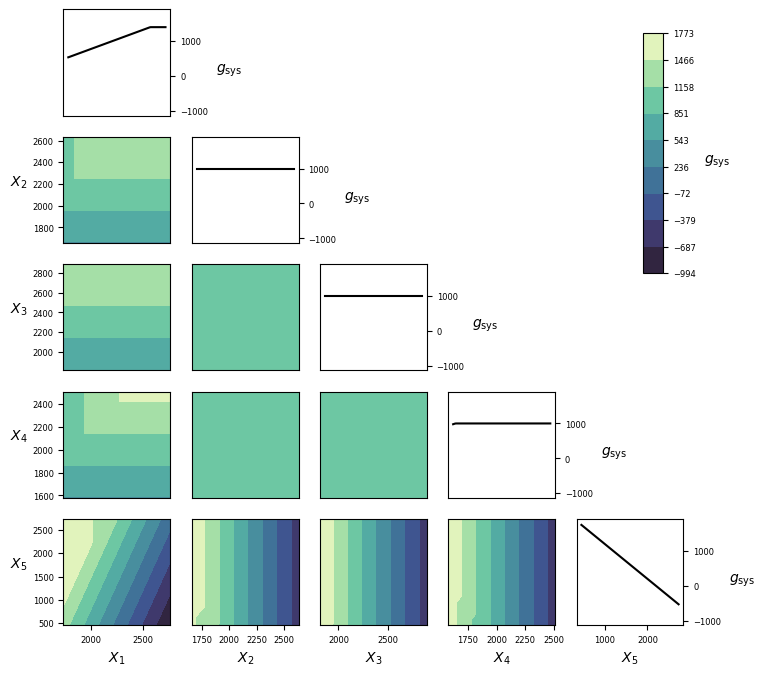### Implementation¶

#### Python¶

gfun_60(x)[source]

Performance function for reliability problem 60.

Parameters: x (numpy.array of float(s)) – Values of independent variables: columns are the different parameters/random variables (x1, x2,…xn) and rows are different parameter/random variables sets for different calls. g_val_sys (numpy.array of float(s)) – Performance function value for the system. g_val_comp (numpy.array of float(s)) – Performance function value for each component. msg (str) – Accompanying diagnostic message, e.g. warning.

## RP63¶

Table 24 – Challenge set 1.
set_id problem_id
1 8

### Overview¶

Category Value
Type Symbolic
Number of random variables 100
Failure probability, $$P_\mathrm{f}$$ $$3.79\cdot10^{-4}$$
Reliability index, $$\beta=-\Phi^{-1}(P_\mathrm{f})$$ 3.36
Number of performance functions 1
Reference [Schueller2004]

### Performance function¶

(16)\eqalign{ & {g_{\mathrm{comp}}}({\bf X}) = 0.1 \cdot \sum\limits_{i = 2}^{100} {X_i^2} - {X_1} - 4.5 \cr & {g_{\mathrm{sys}}}({\bf X}) = {g_{\mathrm{comp}}}({\bf X}) \cr}

### Random variables¶

The parametrization of distributions follows that of in Distributions.

Variable Description Distribution $$\theta_1$$ $$\theta_2$$ $$\theta_3$$ $$\theta_4$$ Mean Std
$$X_1$$ NA Normal 0.0 1.0     0.0 1.0
$$\vdots$$ $$\vdots$$ $$\vdots$$ $$\vdots$$ $$\vdots$$ $$\vdots$$ $$\vdots$$ $$\vdots$$ $$\vdots$$
$$X_{100}$$ NA Normal 0.0 1.0     0.0 1.0

The random variables are mutually independent.

### Visualization¶### Implementation¶

#### Python¶

gfun_63(x)[source]

Performance function for reliability problem 63.

Parameters: x (numpy.array of float(s)) – Values of independent variables: columns are the different parameters/random variables (x1, x2,…xn) and rows are different parameter/random variables sets for different calls. g_val_sys (numpy.array of float(s)) – Performance function value for the system. g_val_comp (numpy.array of float(s)) – Performance function value for each component. msg (str) – Accompanying diagnostic message, e.g. warning.

## RP75¶

Table 25 – Challenge set 1.
set_id problem_id
1 9

### Overview¶

Category Value
Type Symbolic
Number of random variables 2
Failure probability, $$P_\mathrm{f}$$ $$1.07\cdot10^{-2}$$
Reliability index, $$\beta=-\Phi^{-1}(P_\mathrm{f})$$ 2.33
Number of performance functions 1
Reference [Schueller2004]

### Performance function¶

(17)\eqalign{ & {g_{\mathrm{comp}}}({\bf X}) = 3 - {X_1} \cdot {X_2} \cr & {g_{\mathrm{sys}}}({\bf X}) = {g_{\mathrm{comp}}}({\bf X}) \cr}

### Random variables¶

The parametrization of distributions follows that of in Distributions.

Variable Description Distribution $$\theta_1$$ $$\theta_2$$ $$\theta_3$$ $$\theta_4$$ Mean Std
$$X_1$$ NA Normal 0.0 1.0     0.0 1.0
$$X_2$$ NA Normal 0.0 1.0     0.0 1.0

The random variables are mutually independent.

### Visualization¶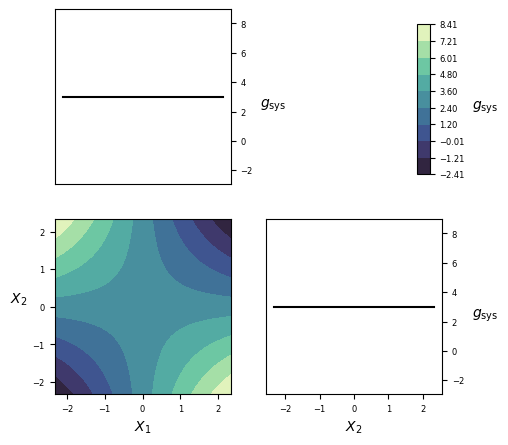### Implementation¶

#### Python¶

gfun_75(x)[source]

Performance function for reliability problem 75.

Parameters: x (numpy.array of float(s)) – Values of independent variables: columns are the different parameters/random variables (x1, x2,…xn) and rows are different parameter/random variables sets for different calls. g_val_sys (numpy.array of float(s)) – Performance function value for the system. g_val_comp (numpy.array of float(s)) – Performance function value for each component. msg (str) – Accompanying diagnostic message, e.g. warning.

## RP77¶

Table 26 – Challenge set 2.
set_id problem_id
2 7

### Overview¶

Category Value
Type Symbolic
Number of random variables 3
Failure probability, $$P_\mathrm{f}$$ $$2.87\cdot10^{-7}$$
Reliability index, $$\beta=-\Phi^{-1}(P_\mathrm{f})$$ 5.00
Number of performance functions 2
Reference [Schueller2004]

### Performance function¶

(18)\eqalign{ & {g_{\mathrm{comp}}}({\bf X}) = \left\{ \matrix{ {X_1} - {X_2} - {X_3}{\rm{ , }}{X_3} \le 5 \hfill \cr {{\rm{X}}_3}{\rm{ - }}{{\rm{X}}_2}{\rm{ , }}{X_3} > 5{\rm{ }} \hfill \cr} \right. \cr & {g_{\mathrm{sys}}}({\bf X}) = {g_{\mathrm{comp}}}({\bf X}) \cr}

### Random variables¶

The parametrization of distributions follows that of in Distributions.

Variable Description Distribution $$\theta_1$$ $$\theta_2$$ $$\theta_3$$ $$\theta_4$$ Mean Std
$$X_{1}$$ NA Normal 10.0 0.5     10.0 0.5
$$X_{2}$$ NA Normal 0.0 1.0     0.0 1.0
$$X_{3}$$ NA Normal 4.0 1.0     4.0 1.0

The random variables are mutually independent.

### Visualization¶

#### Python¶### Implementation¶

gfun_77(x)[source]

Performance function for reliability problem 77.

Parameters: x (numpy.array of float(s)) – Values of independent variables: columns are the different parameters/random variables (x1, x2,…xn) and rows are different parameter/random variables sets for different calls. g_val_sys (numpy.array of float(s)) – Performance function value for the system. g_val_comp (numpy.array of float(s)) – Performance function value for each component. msg (str) – Accompanying diagnostic message, e.g. warning.

## RP89¶

Table 27 – Challenge set 2.
set_id problem_id
2 8

### Overview¶

Category Value
Type Symbolic
Number of random variables 2
Failure probability, $$P_\mathrm{f}$$ $$5.43\cdot10^{-3}$$
Reliability index, $$\beta=-\Phi^{-1}(P_\mathrm{f})$$ 2.55
Number of performance functions 2
Reference [Schueller2004]

### Performance function¶

(19)\eqalign{ & {g_{\mathrm{comp},1}}({\bf X}) = - (X_1^2 + {X_2} - 8) \cr & {g_{\mathrm{comp},2}}({\bf X}) = - \left( {{{{X_1}} \over 5} + {X_2} - 6} \right) \cr & {g_{\mathrm{sys}}}({\bf X}) = \min \left\{ \matrix{ {g_{\mathrm{comp},1}}({\bf X}) \hfill \cr {g_{\mathrm{comp},2}}({\bf X}) \hfill \cr} \right. \cr}

### Random variables¶

The parametrization of distributions follows that of in Distributions.

Variable Description Distribution $$\theta_1$$ $$\theta_2$$ $$\theta_3$$ $$\theta_4$$ Mean Std
$$X_{1}$$ NA Normal 0.0 1.0     0.0 1.0
$$X_{2}$$ NA Normal 0.0 1.0     0.0 1.0

The random variables are mutually independent.

### Visualization¶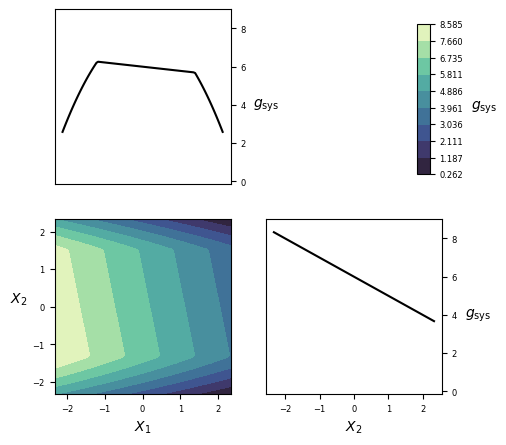### Implementation¶

#### Python¶

gfun_89(x)[source]

Performance function for reliability problem 89.

Parameters: x (numpy.array of float(s)) – Values of independent variables: columns are the different parameters/random variables (x1, x2,…xn) and rows are different parameter/random variables sets for different calls. g_val_sys (numpy.array of float(s)) – Performance function value for the system. g_val_comp (numpy.array of float(s)) – Performance function value for each component. msg (str) – Accompanying diagnostic message, e.g. warning.

## RP91¶

Table 28 – Challenge set 2.
set_id problem_id
2 9

### Overview¶

Category Value
Type Symbolic
Number of random variables 5
Failure probability, $$P_\mathrm{f}$$ $$6.97\cdot10^{-4}$$
Reliability index, $$\beta=-\Phi^{-1}(P_\mathrm{f})$$ 3.19
Number of performance functions 3
Reference [Schueller2004]

### Performance function¶

(20)\eqalign{ & {g_{\mathrm{comp},1}}({\bf X}) = 0.847 + 0.96 \cdot {X_2}{\rm{ + }}0.986 \cdot {X_3} - 0.216 \cdot {X_4} + {\rm{ }}0.077 \cdot X_2^2 + 0.11 \cdot X_3^2 + {{0.007 \cdot X_{_4}^2} \over {0.378}} - {\rm{ }}{X_2} \cdot {\rm{ }}{X_3} - 0.106{\rm{ }} \cdot {X_2} \cdot {X_4} - {\rm{ }}0.11 \cdot {X_3} \cdot {X_4} \cr & {g_{\mathrm{comp},2}}({\bf X}) = {{8400 \cdot {X_1}} \over {\root 2 \of {X_3^2 + X_4^2 - {X_3} \cdot {X_4} + 3 \cdot X_5^2} }} - 1 \cr & {g_{\mathrm{comp},3}}({\bf X}) = {{8400 \cdot {X_1}} \over {\left| {{X_4}} \right|}} - 1 \cr & g({X}) = \min \left\{ \matrix{ {g_{\mathrm{comp},1}}({\bf X}) \hfill \cr {g_{\mathrm{comp},2}}({\bf X}) \hfill \cr {g_{\mathrm{comp},3}}({\bf X}) \hfill \cr} \right. \cr}

### Random variables¶

The parametrization of distributions follows that of in Distributions.

Variable Description Distribution $$\theta_1$$ $$\theta_2$$ $$\theta_3$$ $$\theta_4$$ Mean Std
$$X_{1}$$ NA Normal 0.07433 0.005     0.07433 0.005
$$X_{2}$$ NA Normal 0.1 0.01     0.1 0.01
$$X_{3}$$ NA Normal 13.0 60.0     13.0 60.0
$$X_{4}$$ NA Normal 4751.0 48.0     4751.0 48.0
$$X_{5}$$ NA Normal -684.0 11.0     -684.0 11.0

The random variables are mutually independent.

### Visualization¶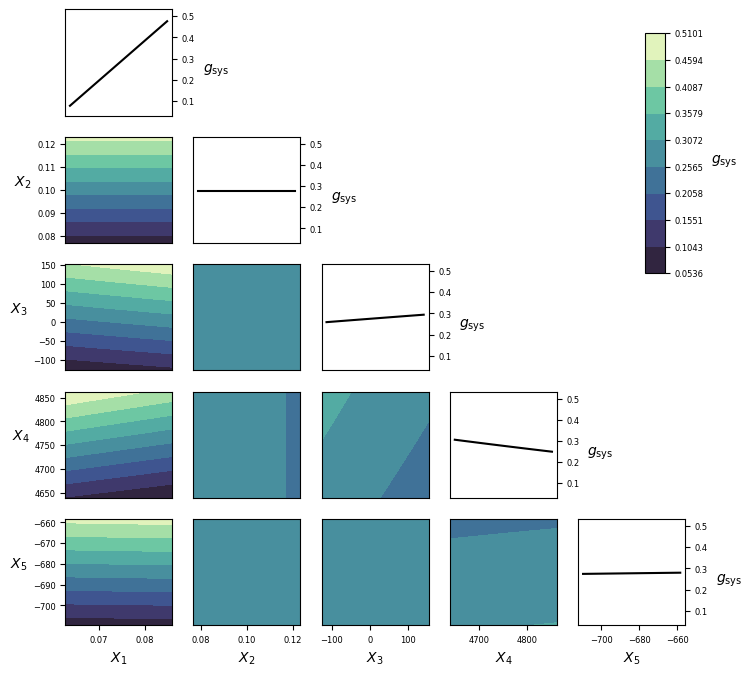### Implementation¶

#### Python¶

gfun_91(x)[source]

Performance function for reliability problem 91.

Parameters: x (numpy.array of float(s)) – Values of independent variables: columns are the different parameters/random variables (x1, x2,…xn) and rows are different parameter/random variables sets for different calls. g_val_sys (numpy.array of float(s)) – Performance function value for the system. g_val_comp (numpy.array of float(s)) – Performance function value for each component. msg (str) – Accompanying diagnostic message, e.g. warning.

## RP107¶

Table 29 – Challenge set 1.
set_id problem_id
1 10

### Overview¶

Category Value
Type Symbolic
Number of random variables 10
Failure probability, $$P_\mathrm{f}$$ $$2.92\cdot10^{-7}$$
Reliability index, $$\beta=-\Phi^{-1}(P_\mathrm{f})$$ 5.0
Number of performance functions 1
Reference [Schueller2004]

### Performance function¶

(21)\eqalign{ & {g_{\mathrm{comp}}}({\bf X}) = 5 \cdot \root 2 \of {10} - \sum\limits_{i = 1}^{10} {{X_i}} \cr & {g_{\mathrm{sys}}}({\bf X}) = {g_{\mathrm{comp}}}({\bf X}) \cr}

### Random variables¶

The parametrization of distributions follows that of in Distributions.

Variable Description Distribution $$\theta_1$$ $$\theta_2$$ $$\theta_3$$ $$\theta_4$$ Mean Std
$$X_1$$ NA Normal 0.0 1.0     0.0 1.0
$$\vdots$$ $$\vdots$$ $$\vdots$$ $$\vdots$$ $$\vdots$$ $$\vdots$$ $$\vdots$$ $$\vdots$$ $$\vdots$$
$$X_{10}$$ NA Normal 0.0 1.0     0.0 1.0

The random variables are identically distributed and mutually independent.

### Visualization¶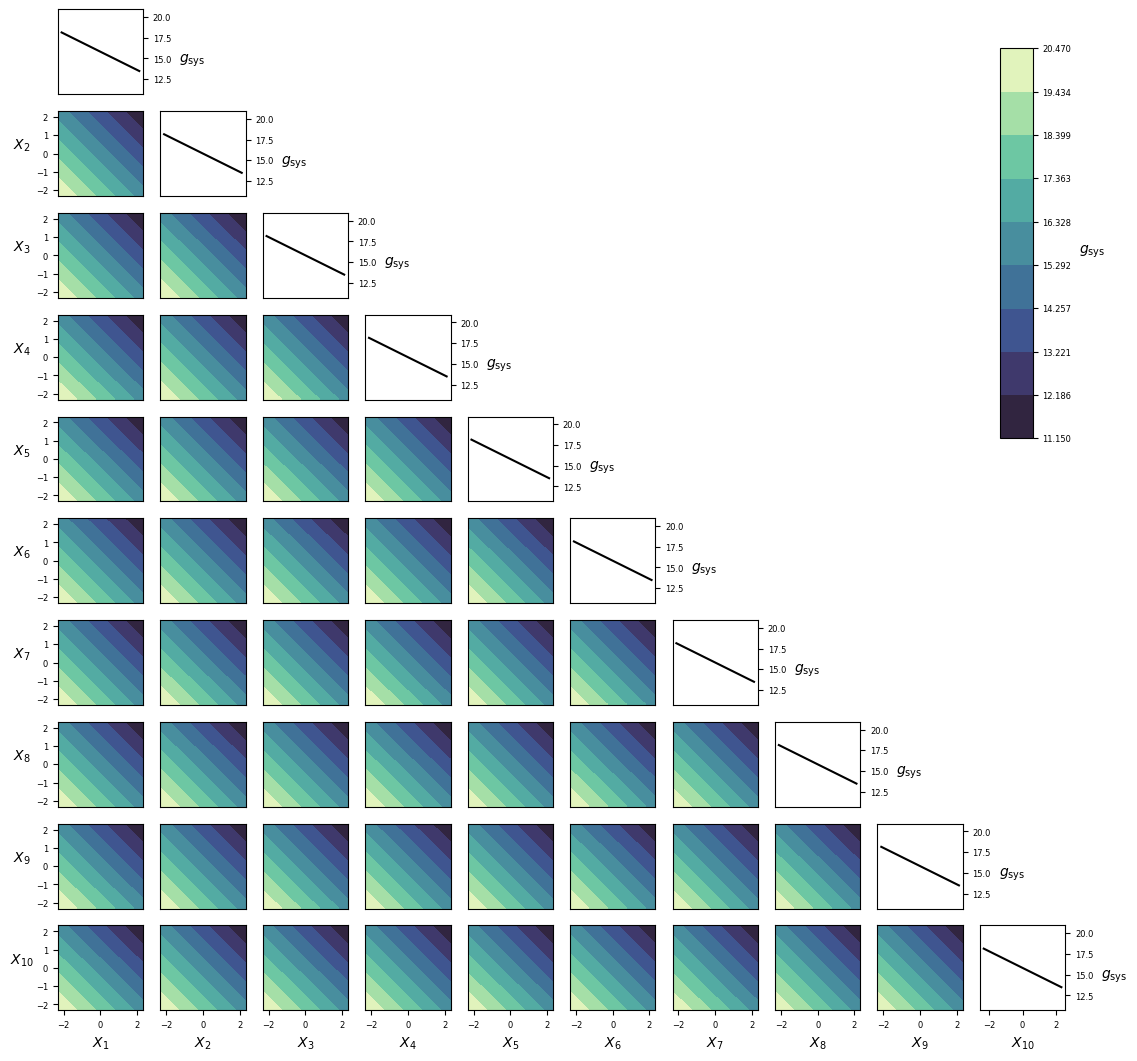### Implementation¶

#### Python¶

gfun_107(x)[source]

Performance function for reliability problem 107.

Parameters: x (numpy.array of float(s)) – Values of independent variables: columns are the different parameters/random variables (x1, x2,…xn) and rows are different parameter/random variables sets for different calls. g_val_sys (numpy.array of float(s)) – Performance function value for the system. g_val_comp (numpy.array of float(s)) – Performance function value for each component. msg (str) – Accompanying diagnostic message, e.g. warning.

## RP110¶

Table 30 – Challenge set 2.
set_id problem_id
2 10

### Overview¶

Category Value
Type Symbolic
Number of random variables 2
Failure probability, $$P_\mathrm{f}$$ $$3.19\cdot10^{-5}$$
Reliability index, $$\beta=-\Phi^{-1}(P_\mathrm{f})$$ 4.00
Number of performance functions 2
Reference [Schueller2004]

### Performance function¶

(22)\eqalign{ & {g_{\mathrm{comp},1}}({\bf X}) = \left\{ \matrix{ 4 - {X_1}{\rm{ , }}{X_1} > 3.5{\rm{ }} \hfill \cr 0.85 - 0.1 \cdot {X_1}{\rm{ , }}{X_1} \le 3.5 \hfill \cr} \right. \cr & {g_{\mathrm{comp},2}}({\bf X}) = \left\{ \matrix{ 0.5 - 0.1 \cdot {X_2}{\rm{ , }}{X_2} > 2 \hfill \cr 2.3 - {X_2}{\rm{ , }}{X_2} \le 2 \hfill \cr} \right. \cr & g({\bf X}) = \min \left\{ \matrix{ {g_{\mathrm{comp},1}}({\bf X}) \hfill \cr {g_{\mathrm{comp},2}}({\bf X}) \hfill \cr} \right. \cr}

### Random variables¶

The parametrization of distributions follows that of in Distributions.

Variable Description Distribution $$\theta_1$$ $$\theta_2$$ $$\theta_3$$ $$\theta_4$$ Mean Std
$$X_{1}$$ NA Normal 0.0 1.0     0.0 1.0
$$X_{2}$$ NA Normal 0.0 1.0     0.0 1.0

The random variables are mutually independent.

### Visualization¶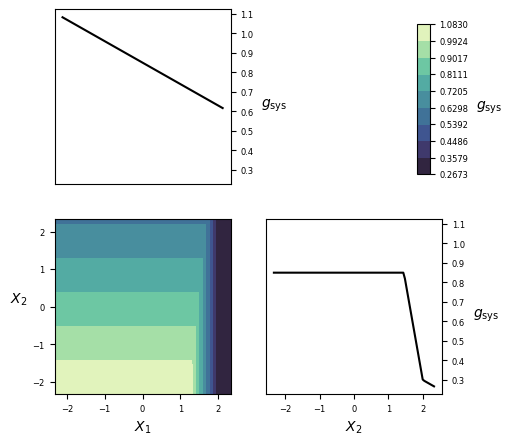### Implementation¶

#### Python¶

gfun_110(x)[source]

Performance function for reliability problem 25.

Parameters: x (numpy.array of float(s)) – Values of independent variables: columns are the different parameters/random variables (x1, x2,…xn) and rows are different parameter/random variables sets for different calls. g_val_sys (numpy.array of float(s)) – Performance function value for the system. g_val_comp (numpy.array of float(s)) – Performance function value for each component. msg (str) – Accompanying diagnostic message, e.g. warning.

## RP111¶

Table 31 – Challenge set 1.
set_id problem_id
1 11

### Overview¶

Category Value
Type Symbolic
Number of random variables 2
Failure probability, $$P_\mathrm{f}$$ $$7.65\cdot10^{-7}$$
Reliability index, $$\beta=-\Phi^{-1}(P_\mathrm{f})$$ 4.81
Number of performance functions 1
Reference [Schueller2004]

### Performance function¶

(23)\eqalign{ & {g_{\mathrm{comp}}}({\bf X}) = {{{5^2}} \over 2} - \left| {{X_1} \cdot {X_2}} \right| \cr & {g_{\mathrm{sys}}}({\bf X}) = {g_{\mathrm{comp}}}({\bf X}) \cr}

### Random variables¶

The parametrization of distributions follows that of in Distributions.

Variable Description Distribution $$\theta_1$$ $$\theta_2$$ $$\theta_3$$ $$\theta_4$$ Mean Std
$$X_1$$ NA Normal 0.0 1.0     0.0 1.0
$$X_2$$ NA Normal 0.0 1.0     0.0 1.0

The random variables are mutually independent.

### Visualization¶### Implementation¶

#### Python¶

gfun_111(x)[source]

Performance function for reliability problem 111.

Parameters: x (numpy.array of float(s)) – Values of independent variables: columns are the different parameters/random variables (x1, x2,…xn) and rows are different parameter/random variables sets for different calls. g_val_sys (numpy.array of float(s)) – Performance function value for the system. g_val_comp (numpy.array of float(s)) – Performance function value for each component. msg (str) – Accompanying diagnostic message, e.g. warning.

## RP201¶

Table 32 – Challenge set 1.
set_id problem_id
1 12

### Overview¶

Category Value
Type Numerical (finite element analysis)
Number of random variables 21
Failure probability, $$P_\mathrm{f}$$ $$1.05\cdot10^{-4}$$
Reliability index, $$\beta=-\Phi^{-1}(P_\mathrm{f})$$ 3.7
Number of performance functions 1
Reference [Blatman2010]

### Performance function¶

Too complex to be written here, see the implementation in the code repository.

### Random variables¶

Variable Description Distribution $$\theta_1$$ $$\theta_2$$ $$\theta_3$$ $$\theta_4$$ Mean Std
$$X_1$$ NA Normal 0.0 1.0     0.0 1.0
$$\vdots$$ $$\vdots$$ $$\vdots$$ $$\vdots$$ $$\vdots$$ $$\vdots$$ $$\vdots$$ $$\vdots$$ $$\vdots$$
$$X_{21}$$ NA Normal 0.0 1.0     0.0 1.0

The random variables are mutually independent.

### Visualization¶### Implementation¶

#### Python¶

gfun_201(x)[source]

Performance function for reliability problem 201.

Parameters: x (numpy.array of float(s)) – Values of independent variables: columns are the different parameters/random variables (x1, x2,…xn) and rows are different parameter/random variables sets for different calls. g_val_sys (numpy.array of float(s)) – Performance function value for the system. g_val_comp (numpy.array of float(s)) – Performance function value for each component. msg (str) – Accompanying diagnostic message, e.g. warning.

## RP202¶

Table 33 – Challenge set 2.
set_id problem_id
2 11

### Overview¶

Category Value
Type Numerical (finite element analysis)
Number of random variables 225
Failure probability, $$P_\mathrm{f}$$ $$6.03\cdot10^{-5}$$
Reliability index, $$\beta=-\Phi^{-1}(P_\mathrm{f})$$ 3.43
Number of performance functions 2
Reference [Schueller2007]

### Performance function¶

Too complex to be written here, see the implementation in the code repository.

### Random variables¶

Variable Description Distribution $$\theta_1$$ $$\theta_2$$ $$\theta_3$$ $$\theta_4$$ Mean Std
$$X_1$$ NA Normal 0.0 1.0     0.0 1.0
$$\vdots$$ $$\vdots$$ $$\vdots$$ $$\vdots$$ $$\vdots$$ $$\vdots$$ $$\vdots$$ $$\vdots$$ $$\vdots$$
$$X_{225}$$ NA Normal 0.0 1.0     0.0 1.0

The random variables are mutually independent.

### Implementation¶

#### Python¶

gfun_202(x)[source]

Performance function for reliability problem 202.

Parameters: x (numpy.array of float(s)) – Values of independent variables: columns are the different parameters/random variables (x1, x2,…xn) and rows are different parameter/random variables sets for different calls. g_val_sys (numpy.array of float(s)) – Performance function value for the system. g_val_comp (numpy.array of float(s)) – Performance function value for each component. msg (str) – Accompanying diagnostic message, e.g. warning.

## RP203¶

Table 34 – Challenge set 1.
set_id problem_id
1 13

### Overview¶

Category Value
Type Numerical (finite element analysis surrogate)
Number of random variables 4
Failure probability, $$P_\mathrm{f}$$ $$4.33\cdot10^{-7}$$
Reliability index, $$\beta=-\Phi^{-1}(P_\mathrm{f})$$ 4.92
Number of performance functions 1
Reference [Eijnden2019]

### Performance function¶

Too complex to be written here, see the implementation in the code repository.

### Random variables¶

The parametrization of distributions follows that of in Distributions.

Variable Description Distribution $$\theta_1$$ $$\theta_2$$ $$\theta_3$$ $$\theta_4$$ Mean Std
$$X_{1}$$ NA Normal 0.0 1.0     0.0 1.0
$$X_{2}$$ NA Normal 0.0 1.0     0.0 1.0
$$X_{3}$$ NA Normal 0.0 1.0     0.0 1.0
$$X_{4}$$ NA Normal 0.0 1.0     0.0 1.0

The random variables are mutually independent.

### Visualization¶### Implementation¶

#### Python¶

gfun_203(x)[source]

Performance function for reliability problem 203. Bram’s geotechnical problem.

Parameters: x (numpy.array of float(s)) – Values of independent variables: columns are the different parameters/random variables (x1, x2,…xn) and rows are different parameter/random variables sets for different calls. g_val_sys (numpy.array of float(s)) – Performance function value for the system. g_val_comp (numpy.array of float(s)) – Performance function value for each component. msg (str) – Accompanying diagnostic message, e.g. warning.

## RP213¶

Table 35 – Challenge set 1.
set_id problem_id
1 14

### Overview¶

Category Value
Type Numeric (finite element analysis)
Number of random variables 13
Failure probability, $$P_\mathrm{f}$$ $$2.84\cdot10^{-4}$$
Reliability index, $$\beta=-\Phi^{-1}(P_\mathrm{f})$$ 3.45
Number of performance functions 1
Reference [Blatman2010]

### Performance function¶

Too complex to be written here, see the implementation in the code repository.

### Random variables¶

Variable Description Distribution $$\theta_1$$ $$\theta_2$$ $$\theta_3$$ $$\theta_4$$ Mean Std
$$X_1$$ NA Normal 0.0 1.0     0.0 1.0
$$\vdots$$ $$\vdots$$ $$\vdots$$ $$\vdots$$ $$\vdots$$ $$\vdots$$ $$\vdots$$ $$\vdots$$ $$\vdots$$
$$X_{13}$$ NA Normal 0.0 1.0     0.0 1.0

The random variables are mutually independent.

### Visualization¶### Implementation¶

#### Python¶

gfun_213_le_frame(x)[source]

Performance function for reliability problem 213.

Parameters: x (numpy.array of float(s)) – Values of independent variables: columns are the different parameters/random variables (x1, x2,…xn) and rows are different parameter/random variables sets for different calls. g_val_sys (numpy.array of float(s)) – Performance function value for the system. g_val_comp (numpy.array of float(s)) – Performance function value for each component. msg (str) – Accompanying diagnostic message, e.g. warning.

## RP300¶

Table 36 – Challenge set 1.
set_id problem_id
1 15

### Overview¶

Category Value
Type Numerical
Number of random variables 14
Failure probability, $$P_\mathrm{f}$$ $$5.22\cdot10^{-5}$$
Reliability index, $$\beta=-\Phi^{-1}(P_\mathrm{f})$$ 3.88
Number of performance functions 1
Reference [Sepulveda2019]

### Performance function¶

Too complex to be written here, see the implementation in the code repository.

### Random variables¶

The parametrization of distributions follows that of in Distributions.

Variable Description Distribution $$\theta_1$$ $$\theta_2$$ $$\theta_3$$ $$\theta_4$$ Mean Std
$$X_1$$ DACC Normal 0 1
$$X_2$$ DACC Normal 0 1
$$\vdots$$ $$\vdots$$ $$\vdots$$ $$\vdots$$ $$\vdots$$
$$X_{14}$$ DACC Normal 0 1

The random variables are mutually independent.

### Visualization¶### Implementation¶

#### Python¶

gfun_300(x)[source]

Performance function for reliability problem 300.

Parameters: x (numpy.array of float(s)) – Values of independent variables: columns are the different parameters/random variables (x1, x2,…xn) and rows are different parameter/random variables sets for different calls. g_val_sys (numpy.array of float(s)) – Performance function value for the system. g_val_comp (numpy.array of float(s)) – Performance function value for each component. msg (str) – Accompanying diagnostic message, e.g. warning.

## RP301¶

Table 37 – Challenge set 1.
set_id problem_id
1 16

### Overview¶

Category Value
Type Numerical (finite element analysis surrogate)
Number of random variables 12
Failure probability, $$P_\mathrm{f}$$ $$6.9\cdot10^{-5}$$
Reliability index, $$\beta=-\Phi^{-1}(P_\mathrm{f})$$ 3.81
Number of performance functions 1
Reference [Slobbe2019]

### Performance function¶

Too complex to be written here, see the implementation in the code repository.

### Random variables¶

The parametrization of distributions follows that of in Distributions.

Variable Description Distribution $$\theta_1$$ $$\theta_2$$ $$\theta_3$$ $$\theta_4$$ Mean Std
$$X_{1}$$ NA Lognormal 4.476 0.05677     88.0 5.0
$$X_{2}$$ NA Lognormal 6.084 0.0681     440.0 30.0
$$X_{3}$$ NA Lognormal 6.18 0.0681     484.0 33.0
$$X_{4}$$ NA Lognormal -2.663 0.08982     0.07 0.0063
$$X_{5}$$ NA Lognormal 6.378 0.06941     590.0 41.0
$$X_{6}$$ NA Lognormal 6.473 0.06925     649.0 45.0
$$X_{7}$$ NA Lognormal -2.663 0.08982     0.07 0.0063
$$X_{8}$$ NA Normal 590.0 59.0     590.0 59.0
$$X_{9}$$ NA Gumbel-max 268.4 46.0     295.0 59.0
$$X_{10}$$ NA Lognormal 0.03797 0.04997     1.04 0.052
$$X_{11}$$ NA Lognormal -0.004975 0.09975     1.0 0.1
$$X_{12}$$ NA Lognormal -0.004975 0.09975     1.0 0.1

The random variables are mutually independent.

### Visualization¶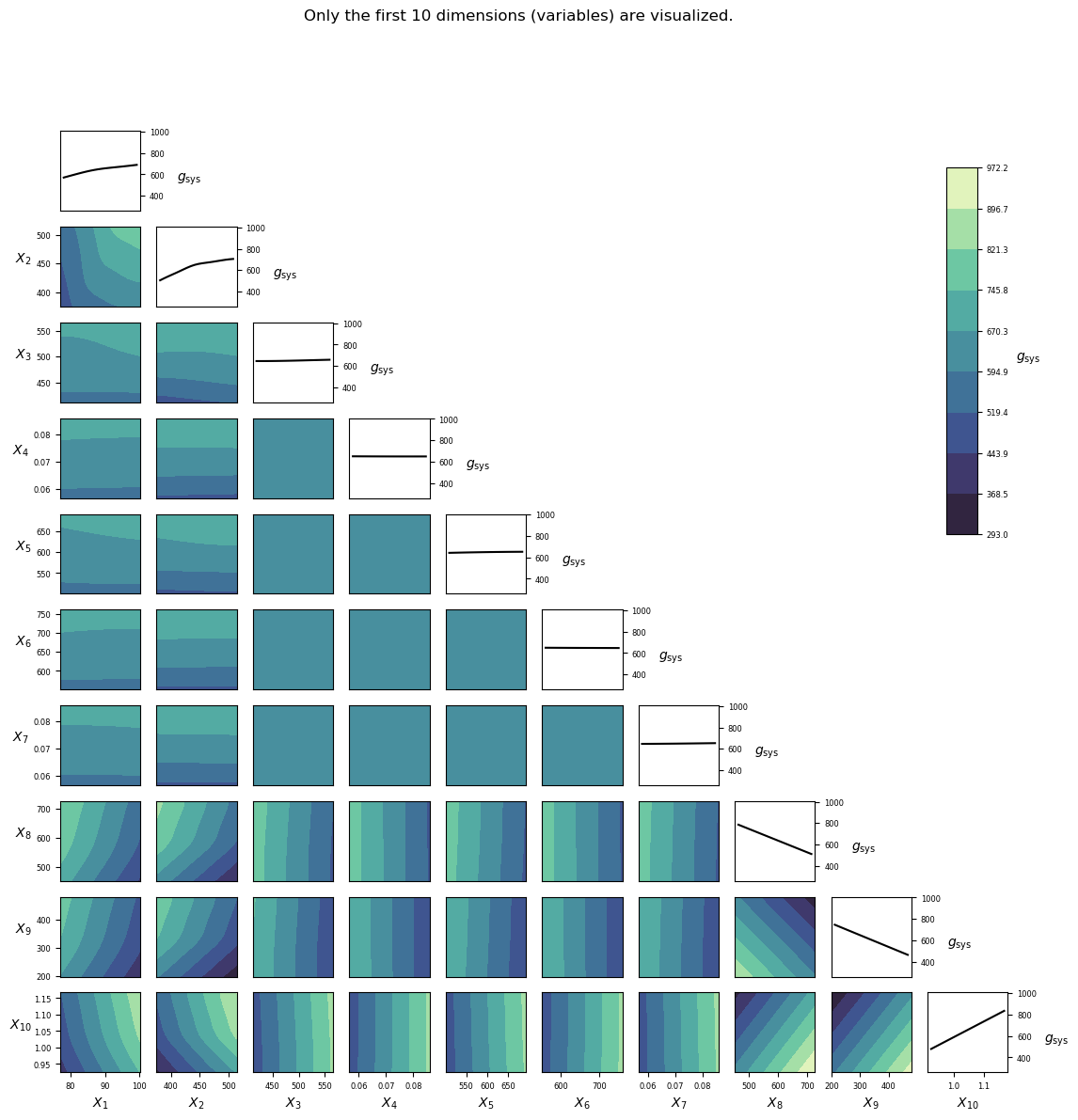### Implementation¶

#### Python¶

gfun_301(x)[source]

Performance function for reliability problem 301. High strength RC deep beam.

Parameters: x (numpy.array of float(s)) – Values of independent variables: columns are the different parameters/random variables (x1, x2,…xn) and rows are different parameter/random variables sets for different calls. g_val_sys (numpy.array of float(s)) – Performance function value for the system. g_val_comp (numpy.array of float(s)) – Performance function value for each component. msg (str) – Accompanying diagnostic message, e.g. warning.